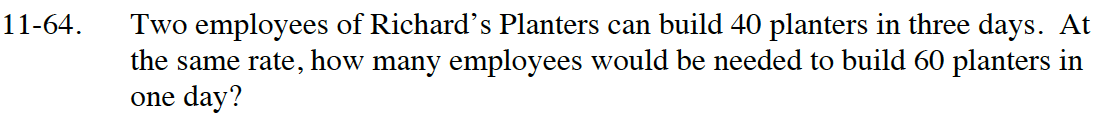### Home > CC4 > Chapter 11 > Lesson 11.2.2 > Problem11-64

11-64.

Two employees of Richard’s Planters can build 40 planters in three days. At the same rate, how many employees would be needed to build 60 planters in one day? Homework Help ✎To find the rate, divide the number of planters by the time it takes to build the planters.

$\text{Rate=\frac{Number\ of\ Planters}{Time}}$

Since there were two original employees, you must divide the rate you found by 2 in order to find the number of planters that 1 employee can make in 1 day.

Let x = the number of employees.
Create an equation such that:
Planters = (Number of Employees)(Rate)

Solve for x.

$\text{Rate=\frac{40}{3}=13.33}$

13.33 ÷ 2 = 6.665 planters per day per employee

60 = x(6.665)

9 employees